# Physical Chemistry : Quantum Numbers

## Example Questions

### Example Question #1 : Quantum Chemistry

Which of the following sets of quantum numbers (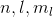) could correspond to a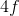orbital?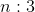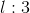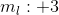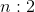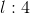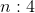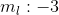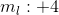Explanation:

Since we're looking at theorbital, we know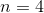. The range of possible values foris 0 to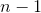. Possible values for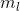range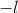to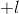. Therefore, among the answer choices,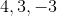is the only possible combination of quantum numbers corresponding to anorbital.

### Example Question #1 : Quantum Chemistry

Which of the following sets of quantum numbers could correspond to a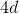orbital?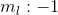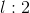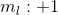Explanation:

Since we're looking at theorbital, we know. The range of possible values foris 0 to. Possible values forrangeto. Therefore, among the answer choices,is the only possible combination of quantum numbers corresponding to aorbital.

### Example Question #3 : Quantum Numbers

What can be concluded about the quantum numbers for potassium and potassium ion?

Valence electron(s) of potassium ion have higher energy

Valence electron(s) of potassium has a higher principal quantum number than valence electron(s) of potassium ion

More than one of these

All valence electron(s) of potassium ion have a different orbital angular momentum quantum number than valence electron(s) of potassium

Valence electron(s) of potassium has a higher principal quantum number than valence electron(s) of potassium ion

Explanation:

Potassium has one valence electron. This means that there is one electron in its outermost shell (4th shell). Potassium ion, on the other hand, loses an electron and has a complete octet (has eight valence electrons) in its 3rd shell. Recall that the principal quantum number signifies the shell. Since the valence electron of potassium is found in the fourth shell,. Similarly, the valence electrons of potassium ion are found in the third shell and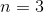for them. Valence electron of potassium has the higher principal quantum number.

Orbital angular momentum number () is the second quantum number and it signifies the type of orbital. It is always greater than or equal to zero.  There are four main types of orbital: s, p, d, and f. Each orbital can hold two electrons. In a given shell, there are one ‘s’ orbital, three ‘p’ orbitals, five ‘d’ orbitals, and seven ‘f’ orbitals. ‘l’ = 0 for ‘s’ orbitals, ‘l’ = 1 for ‘p’ orbitals, ‘l’ = 2 for ‘d’ orbitals, and ‘l’ = 3 for ‘f’ orbitals. In potassium, there is only one valence electron; therefore, there is only one electron in the fourth shell and it can fit into the ‘s’ orbital. In potassium ion, there are eight valence electrons; therefore, two electrons can be found in the ‘s’ orbital and the remaining six electrons can be found in the three ‘p’ orbitals. Not all valence electrons of potassium ion and potassium have different ‘l’ value. This is because valence electron of potassium and two of the valence electrons of potassium ion are found in the ‘s’ orbital (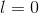).

Electrons found in higher shell numbers have higher energy. Valence electron of potassium is found in the fourth shell; therefore, it will have a higher energy than any of the valence electrons of potassium ion.

### Example Question #4 : Quantum Numbers

Consider the following descriptions of quantum numbers:

A: energy level within a subshell

B: shape of orbital

C: spin of electron

D: energy level

Which of the following is the correct pairing of quantum numbers (1st, 2nd, 3rd, and 4th) with the given descriptions?

A: 4th, B: 2nd, C: 3rd, D: 1st

A: 2nd, B: 3rd, C: 4th, D: 1st

A: 3rd, B: 2nd, C: 4th, D: 1st

A: 3rd, B: 4th, C: 2nd, D: 1st

A: 3rd, B: 2nd, C: 4th, D: 1st

Explanation:

There are four quantum numbers. The first quantum, or principal quantum number, is designated by the letter ‘n’. It signifies the electron shell, or the energy level of the electron. The second quantum number, or orbital angular momentum quantum number, is designated by the letter ‘l’. It signifies the shape (or type) of the orbital. The third quantum number, or magnetic quantum number, is designated by. This signifies the energy level within a subshell. Each orbital can be located in different orientations in space. For example, each of the three ‘p’ orbitals are oriented differently in space and have different energy levels. The third quantum number describes this phenomenon. Finally, the fourth quantum number, or spin quantum number, is designated by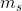and describes the spin of the electron. An electron can spin clockwise or counterclockwise.describes the direction of the spin. Since an electron can only rotate two ways,can only be two values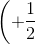or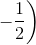.

These four numbers together describe the potential location of an electron inside an atom. Note that no two electrons can have the same set of quantum numbers (meaning at least one of the four numbers will be different).

### Example Question #5 : Quantum Numbers

Which of the following is true regarding ethene? (The electrons in answer choices refer to carbon electrons)

The electrons in the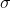bonds and thebond have different principal quantum number

The electrons in thebonds have the same set of quantum numbers

The electrons inbond have the same set of quantum numbers

The electrons in thebond have the same orbital angular momentum number

The electrons in thebond have the same orbital angular momentum number

Explanation:

Ethene, or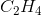, has a carbon-carbon single () and double () bond. Recall that abond can be found in hybridized orbitals whereas abond cannot. This means that the carbon atoms in ethene hybridize the single ‘s’ orbital and two of the ‘p’ orbitals, forming a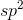hybridization. Thebonds (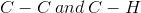) are found in these three hybridized orbitals. The remaining unhybridized ‘p’ orbital will house the two electrons in thebond.

The orbital angular momentum number is the second quantum number and it signifies the type of orbital. An electron found in a ‘p’ orbital will always have an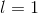. Since both electrons in thebond are found in the ‘p’ orbital, the ‘l’ value for both electrons is the same.

The principal quantum number is the first quantum number and it signifies the shell or energy level of an electron. All electrons involved in bonds are found in carbon’s outermost shell (2nd shell); therefore, they will all have an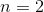. Remember that no two electrons can have the same set of quantum numbers (regardless of whether the electrons are found as lone pairs, inbonds, or inbonds).

### Example Question #6 : Quantum Numbers

Which of the following is/are true regarding the principal quantum number?

I. Principal quantum number signifies the energy level

II. Principal quantum number can never equal zero

III. Principal quantum number can be positive or negative

I and III

II only

I and II

I, II, and III

I and II

Explanation:

Quantum numbers are fancy coordinate systems that describe the potential location of an electron within an atom. The first quantum number is called the principal quantum number and it signifies the shell the electron is located in. Recall that electron shells are discrete orbits in an atom that have discrete energy; therefore, the principal quantum number signifies the energy level of an electron.

The principal quantum number is always an integer and is always greater than zero. If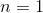the electron is found within the first shell, ifthen the electron is found within the second shell, and so and and so forth. Also, since it is always greater than zero, the principal quantum number can never be negative.

### Example Question #1 : Quantum Numbers

How many subshells are there with n = 4 in an atom?Explanation:

The types of subshells, from smallest to largest, are as follows: s, p, d, and f. These four subshells correspond respectively to the following quantum numbers: 0, 1, 2, and 3. The total number of sublevels with n = 4 is n or 4: 4s, 4p, 4d and 4f.

### Example Question #1 : Quantum Numbers

Which of the following set of quantum numbers is not valid?

n=3

l=2

m=-1

n=2

l=1

m=0

n=3

l=1

m=0

n=4

l=2

m=-3

n=2

l=1

m=1

n=4

l=2

m=-3

Explanation:

The principle quantum number (n) and the angular quantum number (l) are acceptable. However, the magnetic quantum number (m) is restricted to lie between -l and l. Therefore, for l=2, the only possible numbers for m are -2, -1, 0, 1, 2.

### All Physical Chemistry Resources